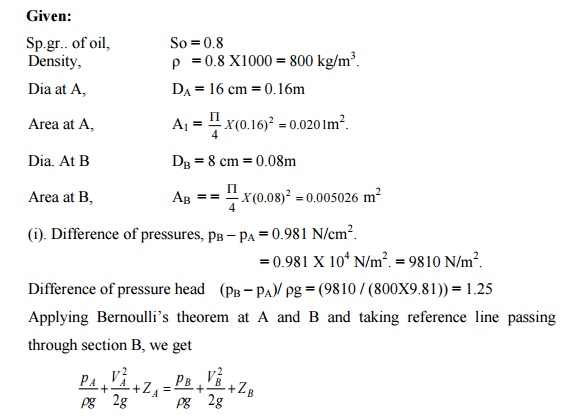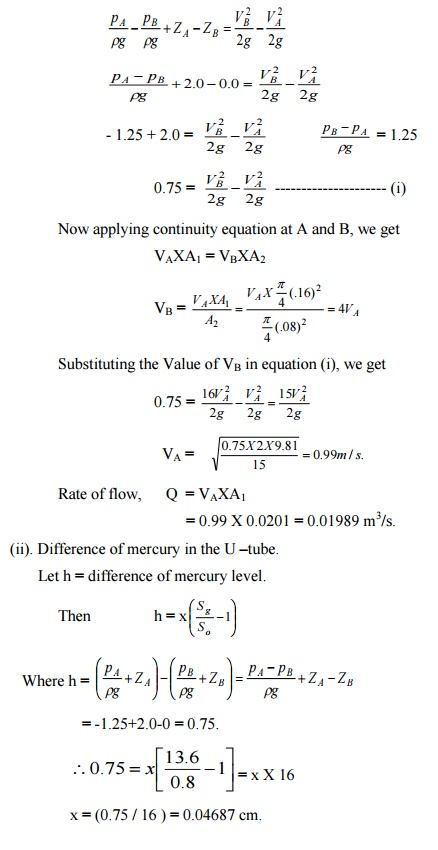# In a vertical pipe conveying oil of specific gravity 0.8, two pressure gauges have been installed at A and B where the diameters are 16 cm and 8 cm respectively. A is 2 m above B. the pressure gauge readings have shown that the pressure at B is greater than at A by 0.981 N/cm2. Neglecting all losses, calculate the flow rate. If the gauges at A and B are replaced by tubes filled with the same liquid and connected to a U – tube containing mercury, calculate the difference of level of mercury in the two limbs of the U-tube.

Question-AnswerCategory: Fluid MechanicsIn a vertical pipe conveying oil of specific gravity 0.8, two pressure gauges have been installed at A and B where the diameters are 16 cm and 8 cm respectively. A is 2 m above B. the pressure gauge readings have shown that the pressure at B is greater than at A by 0.981 N/cm2. Neglecting all losses, calculate the flow rate. If the gauges at A and B are replaced by tubes filled with the same liquid and connected to a U – tube containing mercury, calculate the difference of level of mercury in the two limbs of the U-tube.

In a vertical pipe conveying oil of specific gravity 0.8, two pressure gauges have been installed at A and B where the diameters are 16 cm and 8 cm respectively. A is 2 m above B. the pressure gauge readings have shown that the pressure at B is greater than at A by 0.981 N/cm2. Neglecting all losses, calculate the flow rate. If the gauges at A and B are replaced by tubes filled with the same liquid and connected to a U – tube containing mercury, calculate the difference of level of mercury in the two limbs of the U-tube.